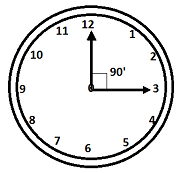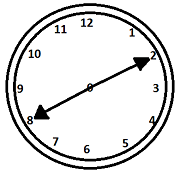# Clock Online Quiz

Following quiz provides Multiple Choice Questions (MCQs) related to Clock. You will have to read all the given answers and click over the correct answer. If you are not sure about the answer then you can check the answer using Show Answer button. You can use Next Quiz button to check new set of questions in the quiz.Q 1 - How much time does a watch gain per day, if its hands coincides in every 64 min.?

A - 37 min.

B - $32\frac{8}{11}$ min

C - 31 min.

D - None of these

### Explanation

$\left ( \frac{720}{11} - x\right )\times\left ( \frac{60\times24}{x} \right )$ min.

Put x = 64, then $32\frac{8}{11}$ min. is correct.

Q 2 - In between 11 and 12 O clock, how many times the two hands will coincide with each other?

A - 60 min.

B - 45 min.

C - 50 min.

D - 55 min.

### Explanation

60 min = 12 hours at which the hands will coincide with each other.

Q 3 - At what time between 8 and 9 Oclock will the hands of a clock be in straight line?

A - 11$\frac{11}{11}$ min past 8

B - 11$\frac{10}{11}$ min past 8

C - 11$\frac{9}{11}$ min past 8

D - 11$\frac{8}{11}$ min past 8

### Explanation

$\left ( \frac{5n - 30}{11} \right )$ × 12 min past (n>6)

$\frac{120}{11}$ = 11 $\frac{10}{11}$ past 8

Q 4 - At what time after 5 Oclock hour hand and the minute hand are at right angle for the first time?

A - 5 hrs 43$\frac{7}{11}$ min

B - 5 hrs 40$\frac{7}{11}$ min

C - 5 hrs 42$\frac{7}{11}$ min

D - 5 hrs 41$\frac{7}{11}$ min

### Explanation

(5n + 15) × $\frac{12}{11}$; n = 5

$\frac{480}{11}$ = 43$\frac{7}{11}$

Q 5 - If two hands are at 15o, they are 15 min space apart. It happens how many times in every 1 hour?

A - Once

B - Twice

C - Thrice

D - None of these

### ExplanationQ 6 - If a clock strikes at 7 Oclock taking 9 sec in order to strike 10 at the same rate what should be the time taken?

A - 81 sec

B - 16 sec

C - 80 sec

D - 14 sec

### Explanation

Time taken for 1 interval = 9 sec

Time taken for 9 intervals = 9 × 9 = 81 sec.

Q 7 - How many times the hands of a clock points towards each other in 24 hours?

A - 20

B - 21

C - 22

D - 23

### Explanation

In general its 11 times points towards each other in 12 hours then in 24 hrs it is double i.e 22 times.

Q 8 - At what time in between 7.30 am to 8.30 am in the morning, the hands are in straight line?

A - $\frac{90}{11}$ min past 7.30

B - $\frac{91}{11}$ min past 7.30

C - $\frac{89}{11}$ min past 7.30

D - $\frac{92}{11}$ min past 7.30

### Explanation$\frac{\left ( 5n - 30 \right ) \times 12}{11}$ (n>6)

$\frac{\left ( 5 \times \left ( \frac{15}{2} \right ) - 30 \right ) \times 12}{11} = \frac{90}{11}$ min past 7.30

Q 9 - How many hours and minutes a watch gains per day, if its hands coincide in every 60 minutes?

A - 130 hrs $\frac{10}{11}$ min

B - 130 hrs $\frac{8}{11}$ min

C - 130 hrs $\frac{9}{11}$ min

D - none of these

### Explanation

$\left ( \frac{720}{11} - x \right ) \times \left ( \frac{60 \times 24}{x} \right )$ min

Here x = 60; $\left ( \frac{720 - 660}{11} \right ) \times\left ( \frac{60 \times 24}{60} \right )$ = 130 hrs$\frac{9}{11}$ min.

Q 10 - The minute hand of a clock overtakes the hour hand at an interval of 63 min of the correct time. How much time does a clock gain or loss in a day?

A - 55$\frac{8}{17}$ min

B - 56$\frac{8}{17}$ min

C - 56$\frac{7}{17}$ min

D - 58$\frac{7}{17}$ min

($\frac{720}{11}$ - x) × ($\frac{60 \times 24}{x}$) min; here x = 63 so, option (b) is correct